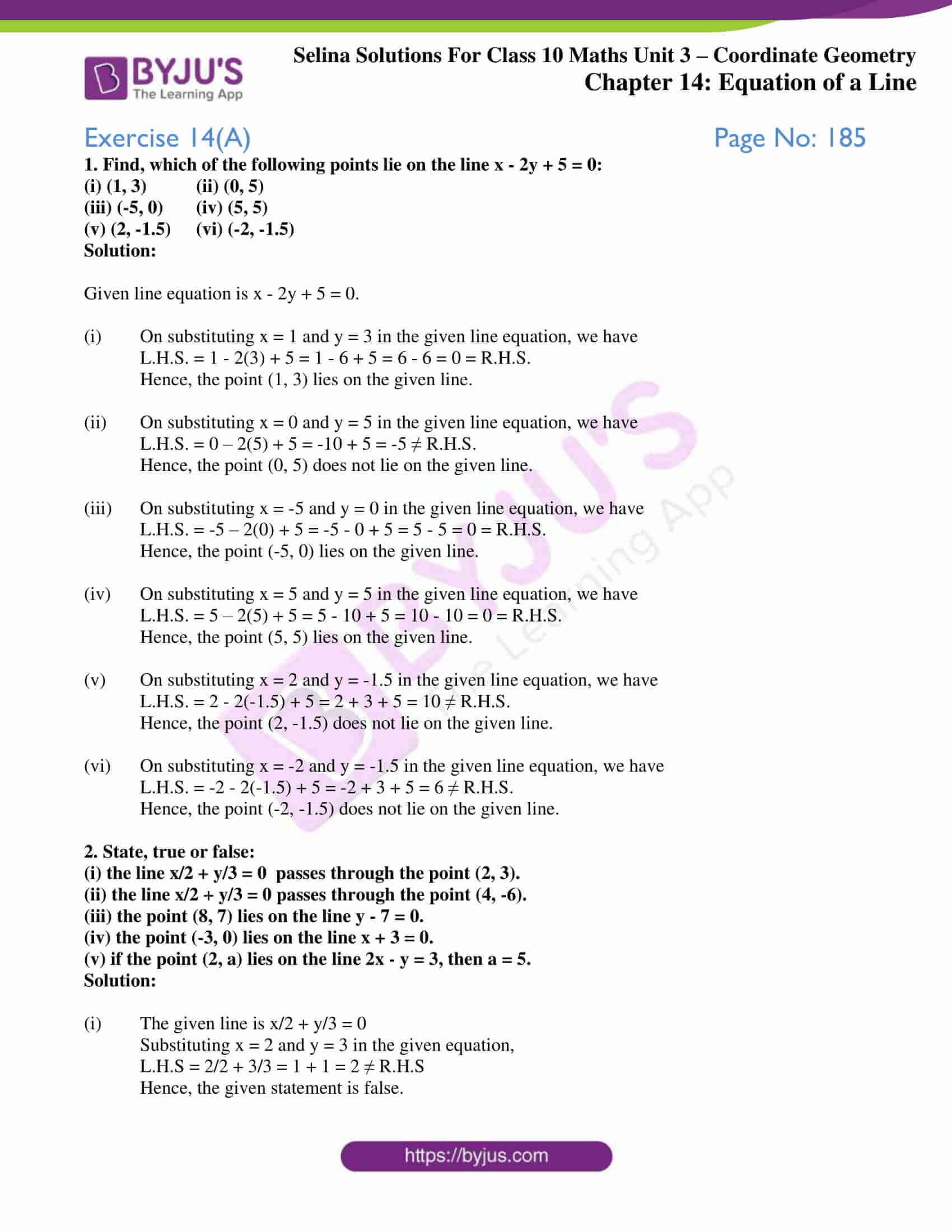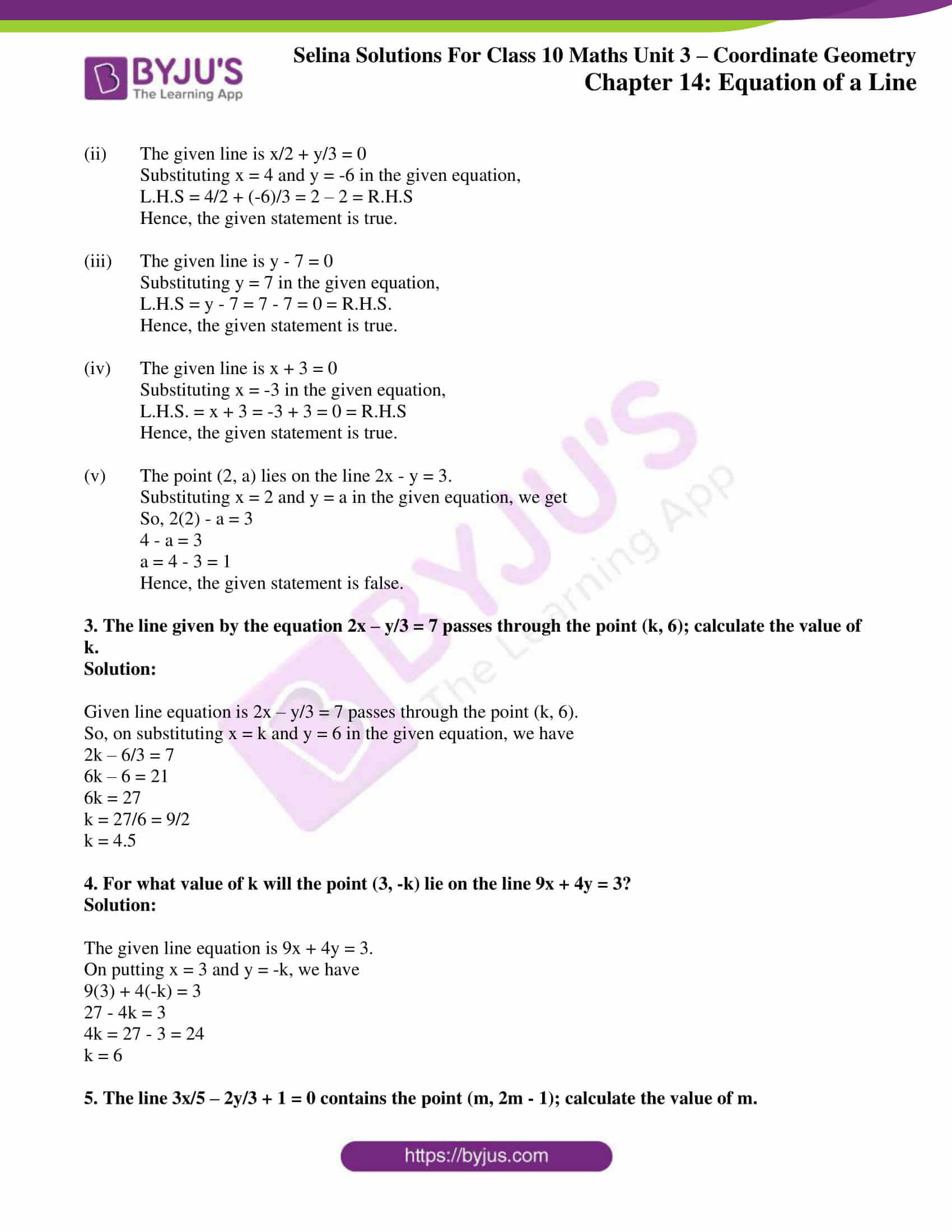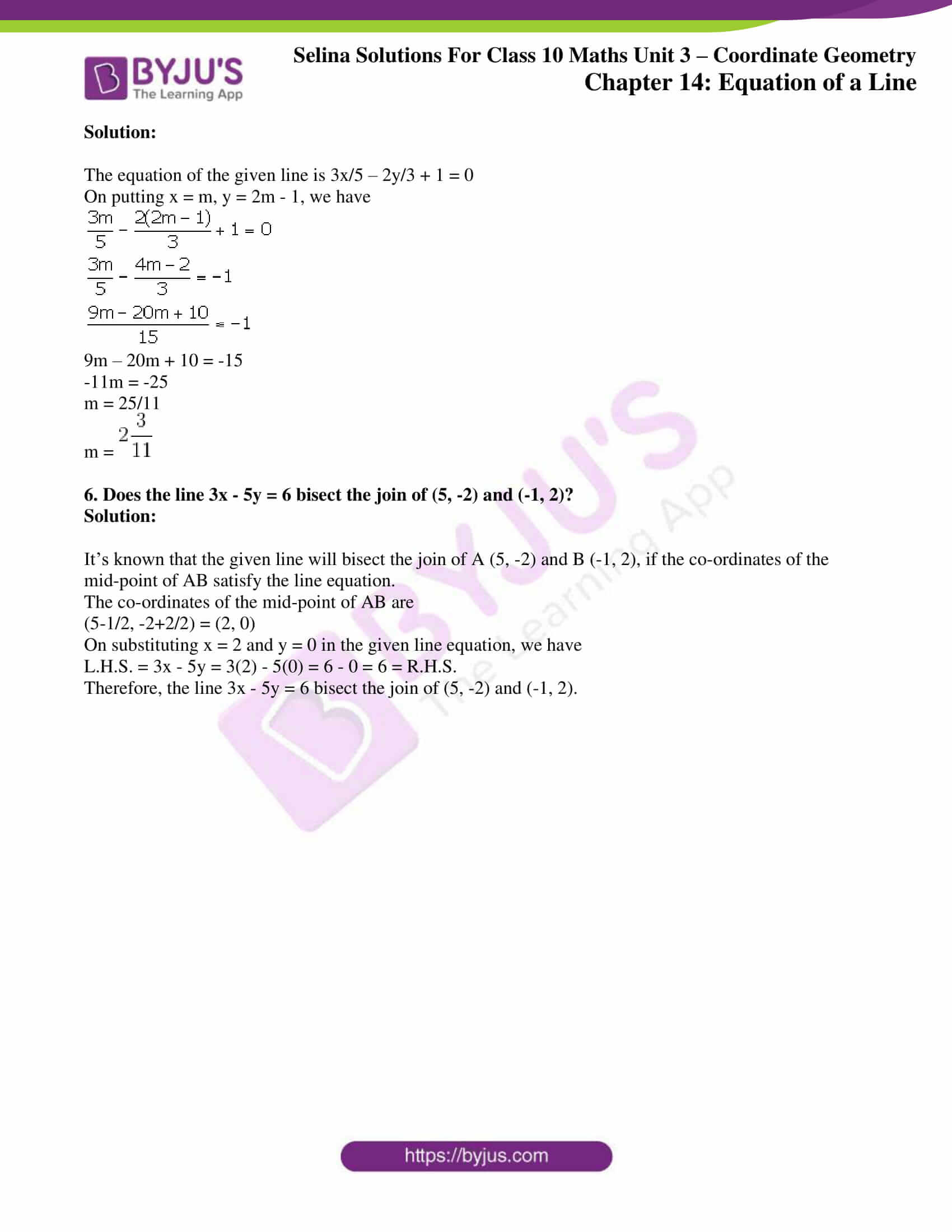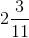# Selina Solutions Concise Maths Class 10 Chapter 14 Equation of a Line Exercise 14(A)

Basic concepts of a line equation like a point satisfying the equation of line and any point, through which a line passes, will satisfy the equation of that line, are understood well by solving these exercise problems. To help students, BYJU”S has created the Selina Solutions for Class 10 Maths where one can refer to the solutions to problems and also clear their doubts. Experts in the subject have created the solutions in accordance with the latest ICSE pattern. Selina Solutions Concise Maths Class 10 Chapter 14 Equation of a Line Exercise 14(A) is available in PDF format, provided in the link below.

## Selina Solutions Concise Maths Class 10 Chapter 14 Equation of a Line Exercise 14(A) Download PDF### Access other exercises of Selina Solutions Concise Maths Class 10 Chapter 14 Equation of a Line

Exercise 14(B) Solutions

Exercise 14(C) Solutions

Exercise 14(D) Solutions

Exercise 14(E) Solutions

### Access Selina Solutions Concise Maths Class 10 Chapter 14 Equation of a Line Exercise 14(A)

#### Exercise 14(A) Page No: 185

1. Find, which of the following points lie on the line x – 2y + 5 = 0:

(i) (1, 3) (ii) (0, 5)

(iii) (-5, 0) (iv) (5, 5)

(v) (2, -1.5) (vi) (-2, -1.5)

Solution:

Given line equation is x – 2y + 5 = 0.

(i) On substituting x = 1 and y = 3 in the given line equation, we have

L.H.S. = 1 – 2(3) + 5 = 1 – 6 + 5 = 6 – 6 = 0 = R.H.S.

Hence, the point (1, 3) lies on the given line.

(ii) On substituting x = 0 and y = 5 in the given line equation, we have

L.H.S. = 0 – 2(5) + 5 = -10 + 5 = -5 ≠ R.H.S.

Hence, the point (0, 5) does not lie on the given line.

(iii) On substituting x = -5 and y = 0 in the given line equation, we have

L.H.S. = -5 – 2(0) + 5 = -5 – 0 + 5 = 5 – 5 = 0 = R.H.S.

Hence, the point (-5, 0) lies on the given line.

(iv) On substituting x = 5 and y = 5 in the given line equation, we have

L.H.S. = 5 – 2(5) + 5 = 5 – 10 + 5 = 10 – 10 = 0 = R.H.S.

Hence, the point (5, 5) lies on the given line.

(v) On substituting x = 2 and y = -1.5 in the given line equation, we have

L.H.S. = 2 – 2(-1.5) + 5 = 2 + 3 + 5 = 10 ≠ R.H.S.

Hence, the point (2, -1.5) does not lie on the given line.

(vi) On substituting x = -2 and y = -1.5 in the given line equation, we have

L.H.S. = -2 – 2(-1.5) + 5 = -2 + 3 + 5 = 6 ≠ R.H.S.

Hence, the point (-2, -1.5) does not lie on the given line.

2. State, true or false:

(i) the line x/2 + y/3 = 0  passes through the point (2, 3).

(ii) the line x/2 + y/3 = 0 passes through the point (4, -6).

(iii) the point (8, 7) lies on the line y – 7 = 0.

(iv) the point (-3, 0) lies on the line x + 3 = 0.

(v) if the point (2, a) lies on the line 2x – y = 3, then a = 5.

Solution:

(i) The given line is x/2 + y/3 = 0

Substituting x = 2 and y = 3 in the given equation,

L.H.S = 2/2 + 3/3 = 1 + 1 = 2 ≠ R.H.S

Hence, the given statement is false.

(ii) The given line is x/2 + y/3 = 0

Substituting x = 4 and y = -6 in the given equation,

L.H.S = 4/2 + (-6)/3 = 2 – 2 = R.H.S

Hence, the given statement is true.

(iii) The given line is y – 7 = 0

Substituting y = 7 in the given equation,

L.H.S = y – 7 = 7 – 7 = 0 = R.H.S.

Hence, the given statement is true.

(iv) The given line is x + 3 = 0

Substituting x = -3 in the given equation,

L.H.S. = x + 3 = -3 + 3 = 0 = R.H.S

Hence, the given statement is true.

(v) The point (2, a) lies on the line 2x – y = 3.

Substituting x = 2 and y = a in the given equation, we get

So, 2(2) – a = 3

4 – a = 3

a = 4 – 3 = 1

Hence, the given statement is false.

3. The line given by the equation 2x – y/3 = 7 passes through the point (k, 6); calculate the value of k.

Solution:

Given line equation is 2x – y/3 = 7 passes through the point (k, 6).

So, on substituting x = k and y = 6 in the given equation, we have

2k – 6/3 = 7

6k – 6 = 21

6k = 27

k = 27/6 = 9/2

k = 4.5

4. For what value of k will the point (3, -k) lie on the line 9x + 4y = 3?

Solution:

The given line equation is 9x + 4y = 3.

On putting x = 3 and y = -k, we have

9(3) + 4(-k) = 3

27 – 4k = 3

4k = 27 – 3 = 24

k = 6

5. The line 3x/5 – 2y/3 + 1 = 0 contains the point (m, 2m – 1); calculate the value of m.

Solution:

The equation of the given line is 3x/5 – 2y/3 + 1 = 0

On putting x = m, y = 2m – 1, we have9m – 20m + 10 = -15

-11m = -25

m = 25/11

m =6. Does the line 3x – 5y = 6 bisect the join of (5, -2) and (-1, 2)?

Solution:

It’s known that the given line will bisect the join of A (5, -2) and B (-1, 2), if the co-ordinates of the mid-point of AB satisfy the line equation.

The co-ordinates of the mid-point of AB are

(5-1/2, -2+2/2) = (2, 0)

On substituting x = 2 and y = 0 in the given line equation, we have

L.H.S. = 3x – 5y = 3(2) – 5(0) = 6 – 0 = 6 = R.H.S.

Therefore, the line 3x – 5y = 6 bisect the join of (5, -2) and (-1, 2).